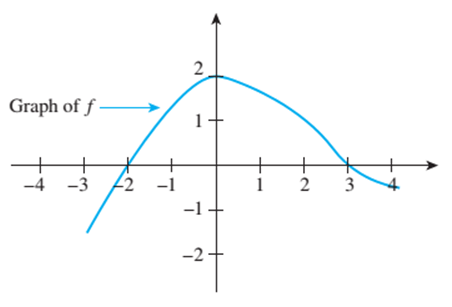Chapter 11.1, Problem 1ES### Discrete Mathematics With Applicat...

5th Edition
EPP + 1 other
ISBN: 9781337694193

#### Solutions

Chapter
Section### Discrete Mathematics With Applicat...

5th Edition
EPP + 1 other
ISBN: 9781337694193
Textbook Problem
1 views

# The graph of a function f is shown below. a. Is f ( 0 ) positive or negative? b. For what values of x does f ( x ) = 0 ? c. Find approximate values for x 1 and x 2 so that f ( x ) = f ( x 2 ) = 1 but x 1 ≠ x 2 . d. Find an approximate value for x such that f ( x ) = 1.5 . e. As x increases from − 3 to − 1 , do the values of f increase or decrease? f. As x increases from 0 to 4, do the values of f increase or decrease?To determine

(a)

Is f(0) positive or negative?

Explanation

Given information:

Concept used:

Value of given function can be determined using graph.

Calculation:

The value of the function f at x=0 is given as f(0)

To determine

(b)

For what values of x does f(x)=0 ?

To determine

(c)

Find approximate values for x1 and x2 so that f(x1)=f(x2)=1 but x1x2.

To determine

(d)

Find an approximate value for x such that f(x)=1.5.

To determine

(e)

As x increases from 3 to 1, do the values of f increase or decrease?

To determine

(f)

As x increases from 0to4, do the values of f increase or decrease?

### Still sussing out bartleby?

Check out a sample textbook solution.

See a sample solution

#### The Solution to Your Study Problems

Bartleby provides explanations to thousands of textbook problems written by our experts, many with advanced degrees!

Get Started